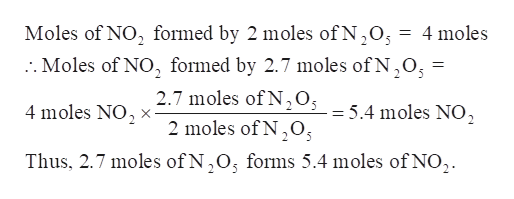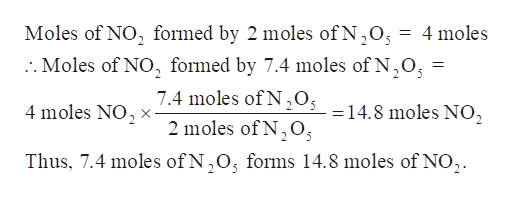# Calculate how many moles of NO2 form when each quantity of reactant completely reacts via the following reaction: 2N2O5(g)→4NO2(g)+ O2(g)2.7 mol N2O57.4 mol N2O515.4 g N2O52.61 kg N2O5

Question
1691 views

Calculate how many moles of NO2 form when each quantity of reactant completely reacts via the following reaction: 2N2O5(g)→4NO2(g)+ O2(g)

2.7 mol N2O5

7.4 mol N2O5

15.4 g N2O5

2.61 kg N2O5

check_circle

Step 1

Number of moles of NO2 form is to be calculated when each quantity of reactant completely reacts via the given reaction:

a) 2.7 mol N2O5

b) 7.4 mol N2O5

c) 15.4 g N2O5

d) 2.61 kg N2O5

Step 2

Part a) 2.7 mol N2O5

As per the balanced equation, 2 moles of N2O5 forms 4 moles of NO2 and 1 mole of O2.help_outlineImage TranscriptioncloseMoles of NO2 formed by 2 moles of N,05 = 4 moles :. Moles of NO, formed by 2.7 moles of N,O, = 2.7 moles of N205 =5,4 moles NO2 2 moles of N,0 4 moles NO X Thus, 2.7 moles of N,O; forms 5.4 moles of NO2. fullscreen
Step 3

Part b) 7.4 mol N2O5

As per the balanced equation, 2 moles of N2O5 forms 4 moles of NO2 and 1 mole of O2.

...help_outlineImage TranscriptioncloseMoles of NO2 formed by 2 moles of N,O :. Moles of NO, formed by 7.4 moles of N,O 4 moles 7.4 moles of N0s =14.8 moles NO, 4 moles NO X 2 moles of N,O Thus, 7.4 moles ofN,O, forms 14.8 moles of NO, fullscreen

### Want to see the full answer?

See Solution

#### Want to see this answer and more?

Solutions are written by subject experts who are available 24/7. Questions are typically answered within 1 hour.*

See Solution
*Response times may vary by subject and question.
Tagged in

### Chemistry